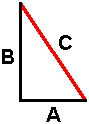Pythagorean Theorem Form - For Hypotenuse Calculate the length of ‘C’ (Hypotenuse of a Right Triangle): The Specs:Length of Side A: (dec.imal or frac.tion) Length of Side B: (dec.imal or frac.tion) The Result Needed: Length of Side C: Legend: 1/32 = 0.03125        1/16 = 0.0625 1/8 = 0.125               3/8 = 0.375 5/8 = 0.625               7/8 = 0.875